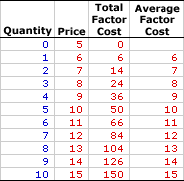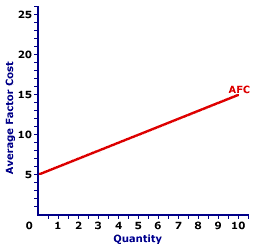Monday  May 29, 2023
 AmosWEB means Economics with a Touch of Whimsy!DEMOGRAPHIC VARIABLES: Characteristics of the aggregate population that marketers use to segment the market, including age, ethnicity, income, education, gender, and race. Other characteristics include occupation, family size, religion, and social class. These characteristics are the link to buyers' wants and needs and affect purchasing behavior. By carefully studying population groups marketers develop marketing mixes that maximize customer activity.AVERAGE FACTOR COST, MONOPSONY:

Total factor cost per unit of factor input employed by a monopsony in the production of output, found by dividing total factor cost by the quantity of factor input. Average factor cost, abbreviated AFC, is generally equal to the factor price. However, using the longer term average factor cost makes it easier to see the connection to related terms, including total factor cost and marginal factor cost.
Average factor cost is the per unit opportunity cost incurred by a monopsony from the employment of a given resource. It plays a key role in the study of factor markets. Average factor cost is constant (that is, it does not change if the quantity of the factor input changes) for a firm buying factors through a perfectly competitive factor market. For a monopsony buyer, average factor cost increases as the quantity of factor input increases. The constant or increasing nature of average factor cost is a prime indication of the market control of a firm.

Whichever market structure is involved, average factor cost is calculated as the total factor cost divided by the quantity of the factor purchased, as illustrated by this equation:

 average factor cost = total factor costfactor quantity
At times, it is useful to turn this equation around and calculate total factor cost from average factor cost:
 total factor cost = average factor cost x factor quantity
If the firm is hiring the factor in a perfectly competitive factor market, then the factor price is fixed or constant and so too is average factor cost. If the firm is hiring the factor in an imperfectly competitive factor market, best illustrated by monopsony, then the factor price increases with larger factor quantities and so too does average factor cost.

Monopsony is a market structure with a single buyer, or in terms of factor markets, a single employer. This means that monopsony is a price maker, with control over the buying side of the market. Market control means monopsony faces a positively-sloped supply curve. To buy a larger quantity, it must pay a higher price.

Average Factor Cost,
MonopsonyThe table to the right summarizes the average factor cost received by a hypothetical firm, OmniKing Island Resort. This firm is the only employer of labor on a small tropical island. As the only employer on the island, OmniKing is a monopsony with extensive market control, and it faces a positively-sloped supply curve. To employ more workers, OmniKing must pay a higher price.

The first column in the table is the quantity of workers hired, ranging from 0 to 10 workers. The second column is the price OmniKing pays for hiring workers, which increases from a low of \$5 for 0 workers to a high of \$15 for 10 workers. The third column is the total factor cost OmniKing incurs for hiring various numbers of workers. If OmniKing hires only one worker, then it pays only \$6. If it hires five workers, it pays \$50. In each case, total factor cost in column three is calculated as the quantity in the first column multiplied by the price in the second column.

The fourth column then presents the average factor cost incurred by OmniKing at each level of employment. These numbers are found by dividing total factor cost in the third column by factor quantity in the first column. For example, the total factor cost of hiring five workers is \$50, resulting in an average factor cost of \$10 per worker.

The two key observations about average factor cost are:

• First, average factor cost is not constant, but increases as the level of employment increases.

• Second, average factor cost is equal to price at every level of employment. Once again, average factor cost and price are basically two terms for the same concept.
Average Factor Cost Curve,
MonopsonyThe average factor cost curve for OmniKing Island Resort is displayed in the exhibit to the right. Key to this curve is that OmniKing is a monopsony buyer of workers and thus faces a positively-sloped supply curve. Larger quantities of input can be had only with higher prices.

The vertical axis measures average factor cost and the horizontal axis measures the quantity of input (workers). Although quantity on this particular graph stops at 10 workers, it could go higher.

This curve indicates that if OmniKing hires 1 worker (at \$6 per worker), then it incurs \$6 of average factor cost. Alternatively, if it hires 10 workers (at \$15 per worker), then it pays \$15 of average factor cost.

This positively-sloped average factor cost curve is actually nothing more than the supply curve facing OmniKing for hiring labor. In the analysis of factor markets, the average factor cost curve and the supply curve facing the firm are almost always one and the same.

Although this average factor cost curve, and preceding table of average factor cost numbers, is based on the employment activity of OmniKing Island Resort, a well-known monopsony firm, they apply to any buyer with market control. Monopsonistic competition and oligopsony firms that also face positively-sloped supply curves generate comparable average factor costs.

 <= AVERAGE FACTOR COST CURVE, PERFECT COMPETITION AVERAGE FACTOR COST, PERFECT COMPETITION =>Recommended Citation:

AVERAGE FACTOR COST, MONOPSONY, AmosWEB Encyclonomic WEB*pedia, http://www.AmosWEB.com, AmosWEB LLC, 2000-2023. [Accessed: May 29, 2023].

Check Out These Related Terms...

Or For A Little Background...

And For Further Study...
Search Again?GRAY SKITTERY[What's This?] Today, you are likely to spend a great deal of time searching for a specialty store trying to buy either a how-to book on home decorating or a set of luggage with wheels. Be on the lookout for bottles of barbeque sauce that act TOO innocent.Your Complete ScopeFrancis Bacon (1561-1626), a champion of the scientific method, died when he caught a severe cold while attempting to preserve a chicken by filling it with snow."The man who does not read good books has no advantage over the man who cannot read them. "-- Mark TwainAR(N)A nth-order Autoregressive ProcessA PEDestrian's Guide Xtra CreditTell us what you think about AmosWEB. Like what you see? Have suggestions for improvements? Let us know. Click the User Feedback link.| | | | | | | | | | |
| | | |

Thanks for visiting AmosWEB